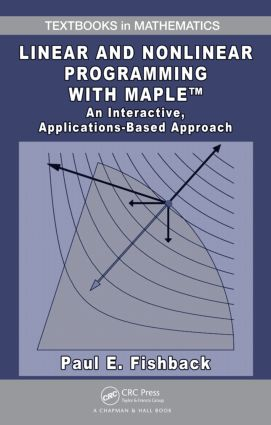# Linear and Nonlinear Programming with Maple

## An Interactive, Applications-Based Approach, 1st Edition

Chapman and Hall/CRC

413 pages | 50 B/W Illus.

##### Purchasing Options:\$ = USD
Hardback: 9781420090642
pub: 2009-12-09
\$120.00
x
eBook (VitalSource) : 9780429126017
pub: 2009-12-09
from \$28.98

FREE Standard Shipping!

### Description

Helps Students Understand Mathematical Programming Principles and Solve Real-World Applications

Supplies enough mathematical rigor yet accessible enough for undergraduates

Integrating a hands-on learning approach, a strong linear algebra focus, Maple™ software, and real-world applications, Linear and Nonlinear Programming with Maple™: An Interactive, Applications-Based Approach introduces undergraduate students to the mathematical concepts and principles underlying linear and nonlinear programming. This text fills the gap between management science books lacking mathematical detail and rigor and graduate-level books on mathematical programming.

Essential linear algebra tools

Throughout the text, topics from a first linear algebra course, such as the invertible matrix theorem, linear independence, transpose properties, and eigenvalues, play a prominent role in the discussion. The book emphasizes partitioned matrices and uses them to describe the simplex algorithm in terms of matrix multiplication. This perspective leads to streamlined approaches for constructing the revised simplex method, developing duality theory, and approaching the process of sensitivity analysis. The book also discusses some intermediate linear algebra topics, including the spectral theorem and matrix norms.

Maple enhances conceptual understanding and helps tackle problems

Assuming no prior experience with Maple, the author provides a sufficient amount of instruction for students unfamiliar with the software. He also includes a summary of Maple commands as well as Maple worksheets in the text and online. By using Maple’s symbolic computing components, numeric capabilities, graphical versatility, and intuitive programming structures, students will acquire a deep conceptual understanding of major mathematical programming principles, along with the ability to solve moderately sized real-world applications.

Hands-on activities that engage students

Throughout the book, student understanding is evaluated through "waypoints" that involve basic computations or short questions. Some problems require paper-and-pencil calculations; others involve more lengthy calculations better suited for performing with Maple. Many sections contain exercises that are conceptual in nature and/or involve writing proofs. In addition, six substantial projects in one of the appendices enable students to solve challenging real-world problems.

### Reviews

"… this text could be ideal for the right course and the right group of students. An independent or directed study in mathematical programming using this book could be an excellent introduction to applied optimization for an interested group of undergraduates. …"

MAA Reviews, March 2010

LINEAR PROGRAMMING

An Introduction to Linear Programming

The Basic Linear Programming Problem Formulation

Linear Programming: A Graphical Perspective in R2

Basic Feasible Solutions

The Simplex Algorithm

The Simplex Algorithm

Alternative Optimal/Unbounded Solutions and Degeneracy

Excess and Artificial Variables: The Big M Method

A Partitioned Matrix View of the Simplex Method

The Revised Simplex Algorithm

Moving beyond the Simplex Method: An Interior Point Algorithm

Standard Applications of Linear Programming

The Diet Problem

Transportation and Transshipment Problems

Basic Network Models

Duality and Sensitivity Analysis

Duality

Sensitivity Analysis

The Dual Simplex Method

Integer Linear Programming

An Introduction to Integer Linear Programming and the Branch and Bound Method

The Cutting Plane Algorithm

NONLINEAR PROGRAMMING

Algebraic Methods for Unconstrained Problems

Nonlinear Programming: An Overview

Differentiability and a Necessary First-Order Condition

Convexity and a Sufficient First-Order Condition

Sufficient Conditions for Local and Global Optimal Solutions

Numeric Tools for Unconstrained Nonlinear Problems

The Steepest Descent Method

Newton’s Method

The Levenberg–Marquardt Algorithm

Methods for Constrained Nonlinear Problems

The Lagrangian Function and Lagrange Multipliers

Convex Nonlinear Problems

Appendix A: Projects

Appendix B: Important Results from Linear Algebra

Appendix C: Getting Started with Maple

Appendix D: Summary of Maple Commands

Bibliography

Index

Exercises appear at the end each section.

### About the Author/Editor

Paul E. Fishback is a professor in the Department of Mathematics at Grand Valley State University in Allendale, Michigan, USA.

### Subject Categories

##### BISAC Subject Codes/Headings:
BUS049000
BUSINESS & ECONOMICS / Operations Research
MAT003000
MATHEMATICS / Applied
TEC029000
TECHNOLOGY & ENGINEERING / Operations Research